Next: Postulates of Quantum Mechanics Up: Mathematical Background Previous: Commutators in Quantum Mechanics

## Linear Vector Spaces in Quantum Mechanics

We have observed that most operators in quantum mechanics are linear operators. This is fortunate because it allows us to represent quantum mechanical operators as matrices and wavefunctions as vectors in some linear vector space. Since computers are particularly good at performing operations common in linear algebra (multiplication of a matrix times a vector, etc.), this is quite advantageous from a practical standpoint.

In an n-dimensional space we may expand any vector
as a linear combination of basis vectors
(80)

For a general vector space, the coefficients ai may be complex; thus one should not be too quick to draw parallels to the expansion of vectors in three-dimensional Euclidean space. The coefficients ai are referred to as the components'' of the state vector, and for a given basis, the components of a vector specify it completely. The components of the sum of two vectors are the sums of the components. If
and
then
(81)

and similarly
(82)

The scalar product of two vectors is a complex number denoted by
(83)

where we have used the standard linear-algebra notation. If we also require that
(84)

then it follows that
(85)

We also require that
(86)

If the scalar product vanishes (and if neither vector in the product is the null vector) then the two vectors are orthogonal.

Generally the basis is chosen to be orthonormal, such that(87)

In this case, we can write the scalar product of two arbitrary vectors as
=(88) =
=
This can also be written in vector notation as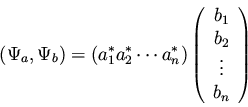(89)

It is useful at this point to introduce Dirac's bra-ket notation. We define a bra'' as
(90)

and a ket'' as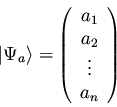(91)

A bra to the left of a ket implies a scalar product, so
(92)

Sometimes in superficial treatments of Dirac notation, the symbol
is defined alternatively as
(93)

This is equivalent to the above definition if we make the connections
and
. This means that our basis vectors are every possible value of x. Since x is continuous, the sum is replaced by an integral (see Szabo and Ostlund  , exercise 1.17). Often only the subscript of the vector is used to denote a bra or ket; we may have written the above equation as
(94)

Now we turn our attention to matrix representations of operators. An operator
can be characterized by its effect on the basis vectors. The action ofon a basis vector
yields some new vector
which can be expanded in terms of the basis vectors so long as we have a complete basis set.
(95)

If we know the effect ofon the basis vectors, then we know the effect ofon any arbitrary vector because of the linearity of.
=
(96) =
or
(97)

This may be written in matrix notation as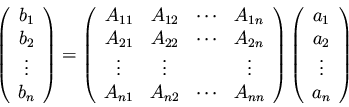(98)

We can obtain the coefficients Aij by taking the inner product of both sides of equation 95 with
, yielding
=
(99) =
= Aij

since
due to the orthonormality of the basis. In bra-ket notation, we may write
(100)

where i and j denote two basis vectors. This use of bra-ket notation is consistent with its earlier use if we realize that
is just another vector
.

It is easy to show that for a linear operator, the inner product
for two general vectors (not necessarily basis vectors)
and
is given by
(101)

or in matrix notation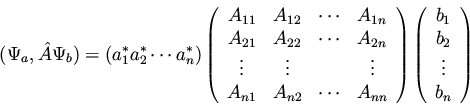(102)

By analogy to equation (93), we may generally write this inner product in the form
(103)

Previously, we noted that, or
. Thus we can see also that
(104)

We now define the adjoint of an operator, denoted by
, as that linear operator for which
(105)

That is, we can make an operator act backwards into bra'' space if we take it's adjoint. With this definition, we can further see that
(106)

or, in bra-ket notation,
(107)

If we pickand
(i.e., if we pick two basis vectors), then we obtain
=
(108)
=Aji* =
But this is precisely the condition for the elements of a matrix and its adjoint! Thus the adjoint of the matrix representation ofis the same as the matrix representation of.

This correspondence between operators and their matrix representations goes quite far, although of course the specific matrix representation depends on the choice of basis. For instance, we know from linear algebra that if a matrix and its adjoint are the same, then the matrix is called Hermitian. The same is true of the operators; if
(109)

thenis a Hermitian operator, and all of the special properties of Hermitian operators apply toor its matrix representation.Next: Postulates of Quantum Mechanics Up: Mathematical Background Previous: Commutators in Quantum Mechanics